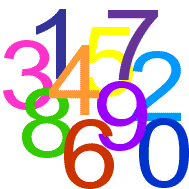#### You may also like### DOTS Division

Take any pair of two digit numbers x=ab and y=cd where, without loss of generality, ab > cd . Form two 4 digit numbers r=abcd and s=cdab and calculate: {r^2 - s^2} /{x^2 - y^2}.### Novemberish

a) A four digit number (in base 10) aabb is a perfect square. Discuss ways of systematically finding this number. (b) Prove that 11^{10}-1 is divisible by 100.### Latin Numbers

Can you create a Latin Square from multiples of a six digit number?

# Number Rules - OK

##### Age 14 to 16 Challenge Level:Can you convince me of each of the following?

• The pattern below continues forever: $$8^2 = 7^2 + 7 + 8$$ $$9^2 = 8^2 + 8 + 9$$
• If a square number is multiplied by a square number the product is ALWAYS a square number.
• No number terminating in $2, 3, 7$ or $8$ is a perfect square.